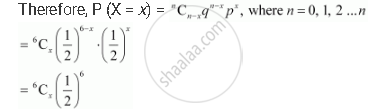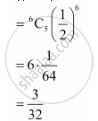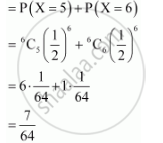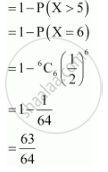Share

# A Die is Thrown 6 Times. If ‘Getting an Odd Number’ is a Success, What is the Probability of - CBSE (Science) Class 12 - Mathematics

ConceptBernoulli Trials and Binomial Distribution

#### Question

A die is thrown 6 times. If ‘getting an odd number’ is a success, what is the probability of

(i) 5 successes?

(ii) at least 5 successes?

(iii) at most 5 successes?

#### Solution

The repeated tosses of a die are Bernoulli trials. Let X denote the number of successes of getting odd numbers in an experiment of 6 trials.

Probability of getting an odd number in a single throw of a die is p = 3/6 =1/2

:. q = 1 - p=1/2

X has a binomial distribution.(i) P (5 successes) = P (X = 5)(ii) P(at least 5 successes) = P(X ≥ 5)(iii) P (at most 5 successes) = P(X ≤ 5)Is there an error in this question or solution?

#### Video TutorialsVIEW ALL 

Solution A Die is Thrown 6 Times. If ‘Getting an Odd Number’ is a Success, What is the Probability of Concept: Bernoulli Trials and Binomial Distribution.
S